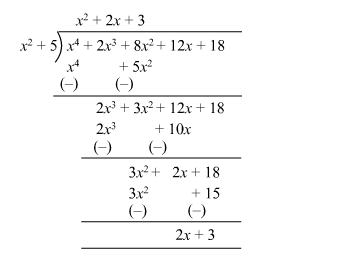# If the polynomial

Question:

If the polynomial $\left(x^{4}+2 x^{3}+8 x^{2}+12 x+18\right)$ is divided by another polynomial $\left(x^{2}+5\right)$, the remainder comes out to be $(p x+q)$. Find the values of $p$ and $q$.

Solution:

Let $f(x)=x^{4}+2 x^{3}+8 x^{2}+12 x+18$

It is given that when $f(x)$ is divisible by $x^{2}+5$, the remainder comes out to be $p x+q$.On division, we get the quotient $x^{2}+2 x+3$ and the remainder $2 x+3$

Since, the remainder comes out to be px + q.

Therefore, = 2 and q = 3.

Hence, the values of p and are 2 and 3 respectively.